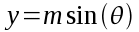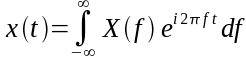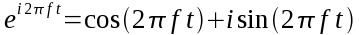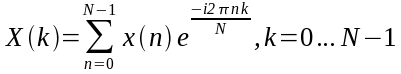Home | Mathematics | Signal Processing | * Signal Processing Workshop 2. The Discrete Fourier Transform 3. The Fast Fourier TransformShare This Page
The Discrete Fourier Transform

A practicum on Fourier analysis and signal processing

Complex Numbers and Vectors | Fourier Basics | Discrete Steps of Time and Frequency
The Nyquist Criterion | The DFT in C++ | Discussion
References

(double-click any word to see its definition)

Complex Numbers and Vectors

Before describing the Fourier Transform, we need to describe some mathematical notation conventions. The data processing methods described in this article depend on the use of "complex numbers," that is, numbers having two orthogonal components (components separated by 90°). One way of interpreting these numbers is as having "real" and "imaginary" components, but this convention is only one way to describe what are in fact two-dimensional Cartesian vectors, numbers that have both magnitude and direction (contrast this with a scalar, a number with only a magnitude).

The "complex number" convention has certain computational advantages, and for this convention, a number pair (x,y) is written like this:

x + iy

Where i = the square root of -1. As it turns out, multiplying a number by i has the effect of rotating it through 90 degrees, so this operation provides the expected 90° relation between the components.

Regardless of whether one thinks of these number pairs as "real" and "imaginary", or "x" and "y" points on a Cartesian plane, it is important to understand that the various notational conventions are interchangeable — they are equivalent descriptions of a two-dimensional vector.

There is even a third way to describe a vector — the "polar notation". Consider this diagram:In this diagram, we have:

• x = the rectangular "horizontal" component of a Cartesian vector.
• y = the rectangular "vertical" component.
• m = the polar "magnitude" of the vector.
• θ = the polar angle of the vector.
One can obtain the polar form (m,θ) of this vector from the rectangular (x,y), and vice versa, using these conversions:
• Rectangular to Polar (x,y) -> (m,θ):

••• Polar to Rectangular (m,θ) -> (x,y):

••In signal processing work, complex numbers and vectors are ubiquitous, so it is a good idea to become comfortable with their various representations. We've just described three vector representations, all equivalent, with different best uses — complex (x+iy), rectangular (x,y) and polar (m,θ).

It is also useful to visualize vectors in particular ways — for example, a vector that represents a time-varying sinewave can be thought of as the spinning hand of a clock, with a full sinewave cycle represented by a complete rotation of the hand. Look at the vector diagram above and imagine the green m "magnitude" line rotating around the (0,0) point, and think about the resulting x and y values. A bit of thought and visualization like this can help one imagine the result of multiplying two vectors together, an operation at the heart of the Fourier Transform.

Fourier Basics

In signal processing, the primary operation is conversion between time and frequency domains. The Fourier Transform (FT) converts a time-varying function into the frequency domain, and the Inverse Fourier Transform (IFT) converts a frequency-domain function into the time domain. Not unlike derivation and integration in Calculus, the FT and IFT are reciprocal operations — all things being equal, the IFT of an FT should produce the original function or data set.

The Fourier Transform (conversion from the time domain to the frequency domain) is defined as:While the Inverse Fourier Transform (frequency to time domain) is defined as:Where:

• i represents the square root of -1.
• f represents frequency in Hertz.
• t represents time in seconds.
• x(t) represents a complex function in the time domain.
• X(f) represents a complex function in the frequency domain.

As it turns out and fortunately, the following relation holds (Euler's Formula):And therefore:It is only because of the relation expressed in Euler's Formula that we can write practical tools based on the Fourier Transform.

Discrete Steps of Time and Frequency

Because we would like to apply these ideas to a signal source rather than a mathematical function, we will now examine the Discrete Fourier Transform (DFT), a method that can be applied to a collection of real-world data points. The Discrete Fourier Transform (DFT) (time domain to frequency domain) is defined as:While the Inverse Discrete Fourier transform (IDFT) (frequency domain to time domain) is defined as:Where:

• x(n) is an array of complex time-domain data.
• n is an index of time steps.
• X(k) is an array of complex frequency-domain data.
• k is an index of frequency spectral lines.
• N represents the size of the data arrays.

It may not be clear to the nontechnical reader, but the DFT and IDFT expressions above require nested loops, one for n and one for k, as a result the solution time increases as O(N2), consequently this method is not practical for most real-world data sets. The value of the DFT lies, not in processing data, but in clarifying technical issues.

The Nyquist Criterion

The DFT differs from the classical Fourier Transform in that it deals with non-continuous data, data composed of discrete points along a time or frequency interval. This is in many ways an advantage (we can use real-world data sets) but it includes a very important limitation called the Nyquist–Shannon sampling theorem: to properly analyze time-varying data with a maximum frequency of F, we need to acquire samples at twice that rate, or 2F. This is an important rule of thumb for any discrete data sets or sources that have bandwidth limitations.

The DFT in C++

Listed below is a working embodiment of the DFT written in C++. Remember it is very slow, not practical for serious processing work. Also note that the arrays "input_data" and "output_data" contain complex numbers. This code's purpose is only to show the conversion process — it is meant more for a reader than a computer, although it works for both.

void DFT::dft1(bool inverse)
{
double pi2 = (inverse)?2.0 * M_PI:-2.0 * M_PI;
double a,ca,sa;
double invs = 1.0 / size;
for(unsigned int y = 0;y < size;y++) {
output_data[y] = 0;
for(unsigned int x = 0;x < size;x++) {
a = pi2 * y * x * invs;
ca = cos(a);
sa = sin(a);
output_data[y].re += input_data[x].re * ca - input_data[x].im * sa;
output_data[y].im += input_data[x].re * sa + input_data[x].im * ca;
}
if(!inverse) {
output_data[y] *= invs;
}
}
}

NOTE: This code example is part of the source code package for this article, where it appears in context, fully operational, and really slow.

Discussion

To those familiar with computer programming, it will be apparent from the C++ listing above that each element in the output array is provided with a summation of all data elements in the input array, each sequentially multiplied by a rotating vector whose angular velocity corresponds to a particular frequency.

At this point I ask my readers to recall an earlier suggestion to visualize a vector as the spinning hand of a clock. In the above code, for each desired frequency we create a vector that "rotates" at that frequency, and multiply the vector by a sequence of data points that may or may not contain the reference frequency. If the data set contains the desired frequency as one of its components, that component will match the reference vector's rotation rate and will appear in the output as a spectral line.

Notwithstanding its conceptual clarity, it will be apparent that the DFT algorithm is very inefficient, requiring two trigonometric computations and several complex operations per data point, and a total of N2 such data points.

Speed optimizations for this routine might include writing in C rather than C++ (less significant as the years go by and compilers become more sophisticated in their optimizations), or using separate arrays for real and imaginary values instead of a complex number class. Or one could simply replace it with a Fast Fourier Transform (FFT), our next topic.

References

 Home | Mathematics | Signal Processing | * Signal Processing Workshop 2. The Discrete Fourier Transform 3. The Fast Fourier TransformShare This Page